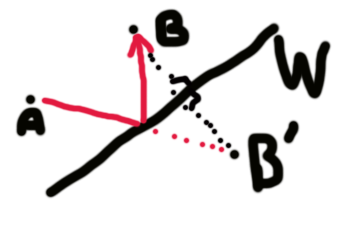# Finding the angle to bounce object to target

How would I work out what angle an object like a pool ball would need to be hit in order for it to bounce off a wall and then hit the required target. I’m currently just finding the half way point between ball and target for one axis and the walls position for the other but this is not accurate enough. I assume it’s a simple maths equation but I’ve had no look in finding it.

So, you have a ball A, a ball B and a wall W. You want the ball A to bounce off the wall W and hit B. Assuming no sliding, spinning, etc, simply reflect the ball B about the wall to a new position B’. Then the point where A->B’ intersects the wall is where to aim for.